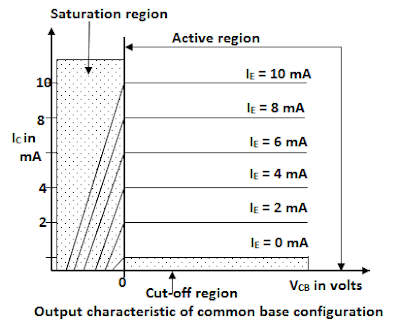# Common Base Configuration

Consider a n-p-n transistor in common base configuration. In this common base configuration, emitter current IE is the input and collector current IC is the output current.# Common Base Configuration

#### Current Amplification Factor (α)

The ratio of the transistor output current to the input current is called current gain of a transistor.
The ration of the collector current to the emitter current is called dc forward current transfer ratio or the dc current gain. It is designated by αdc  and is known as the alpha dc.

Common Base dc current gain, αdc = IC / IE.
Where IC and IE are the magnitude of the collector current and emitter currents at a particular point on the transistor characteristics.

As the collector current is always less than emitter current, the value of αdc is always less than unity.
Typical values of αdc lies in the range of 0.95 to 0.998.
From equation, αdc = IC / IE, We have IC = αdc IE.
We can write αdc simply as α, then IC =αIE
Since IC = IC(majority) + ICBO(minority), the above equation can be written as
IC = α IC (majority) + ICBO
We know that IE = IC + Ior

IB = IE – IC  = IE – (αIE + ICBO)

IB = IE (1-α) – ICBO
Neglecting ICBO, we can write IB = IE (1-α)
Common-base short circuit (ac) current gain is defined as the ratio of a small change in collector current (ΔIC) to the corresponding change in emitter current (ΔIE) at constant collector-base voltage. It is denoted by αac.
αac = (ΔIC / ΔIE) / VCB = constant.

For all practical purpose dc current gain is considered equal to the ac current gain. i.e. αdc = αac.
This parameter is extremely important in transistor theory. It should be pointed out that α is not a constant, but varies with emitter current IE, collector to base voltage VCB and temperature.
• It may be note that the current gain of a transistor in common-base configuration is less than unity. But still it is called the current gain; It is due to the fact that the output resistance of common-base transistor is much higher than the input resistance. This produces a large voltage gain and hence the large power gain.

## Characteristics of Common Base Configuration• The figure above shows that the experimental arrangement for determining the static characteristic of an n-p-n transistor in common-base mode.
• Two regulated dc power supplies VEE and VCC are connected in the circuit as shown in the fig. two milli-ammeters and two voltmeters are included in the circuit to not the required current and voltage to draw characteristic.

### Input Characteristics of common base configuration (VEE vs IE, VCB = Constant)• Let the collector to base voltage VCB (say 2V) be kept constant The emitter to base voltage VEB is varied in small steps, say 0.1s repeated V and the corresponding values of emitter current IE are noted for each value of VEB.
• The test is repeated for various values of VCB. It is found that the increasing levels of VCB results in a reduced level VEB to establish the same current.
• The input characteristic of a typical transistor of common-base mode are shown if fig. the emitter current IE is taken along Y axis and emitter-base voltage VEB along X axis. Note the tight grouping of the curves for the wide range of values for VCB. The average value of the curve appear to begin its rise at about VEB=0.5 for Si transistor a 25oC.
• The emitter currents are almost independent of VCB. As with the semiconductor silicon diode, a first approximation for the forward-biased base-emitter junction in the dc mode would that VEB = 0.7V.

### Output Characteristic of common base configuration (VCB Vs IC, IE = Constant)

The emitter current, IE is kept constant (say 2mA). The collector to base voltage is varied from zero in suitable steps; say IV and corresponding values of IC are noted. The experiment is repeated for different values of IE. The output characteristic curves obtained are shown in fig.The common base configuration output characteristics can be divided into three distinct regions namely
1. Active region
2. saturation region
3. cut-off region
• The active region is the region that is located to the right of the line VCB=0 and above the emitter current, IE=0. In this region, the collector current is constant and is almost equal to the emitter current.
• The saturation region id the region that located to the left of the line VCB=0 and above the output characteristic if emitter current IE=0. The collector current, IC increases sharply for a small change in VCB.
• In the above figure the emitter current increases above zero, the collector current increases to a magnitude equal to that of the emitter current as determined by the basic transistor-current relations. The curves clearly indicate that a first approximation to the relationship between IC and IE in the active region is give by IC=IE.
• When IE=0, IC=ICBO, which is the leakage current of the collector base diode. IE=0 is called cut-off region.
The output characteristic curve may be used to determine dynamic output resistance, It is defined as the ration of a small change in collector to base voltage to the corresponding change in collector current are constant emitter current.
output Resistance Ro = {ΔVCB / ΔIC} IE = Constant

• The reciprocal of the slope of the output characteristics gives the dynamic output resistance. The value of Ro is very high and is of the order of mega ohms.
• The characteristic may be used to determine small-signal common-base current gain or ac alpha (αac) of transistor. This can be done by selecting two points M and N on the characteristic and note down the corresponding values of ΔIC and ΔIE.
αdc = ΔIC / ΔIE  = 2mA / 2mA = 1 mA0 CommentsComments